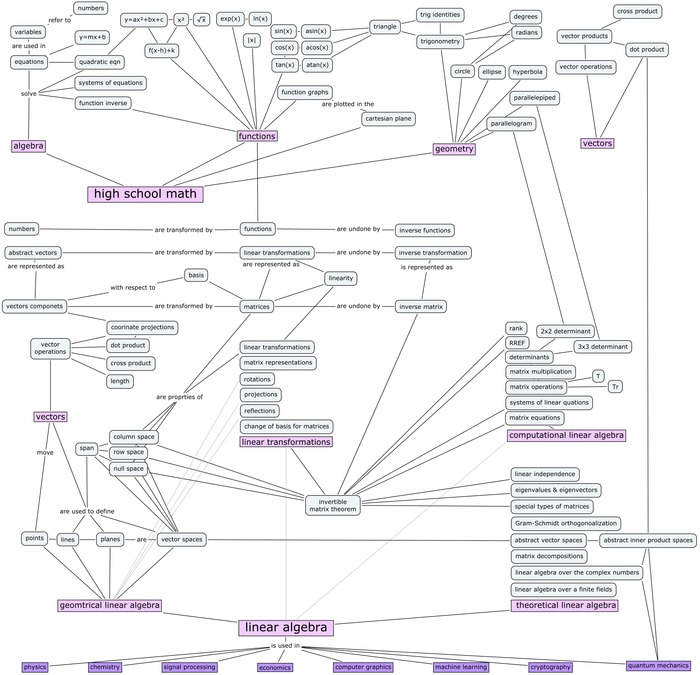# Linear Algebra

Tue 29 May 2018

## Vectors

Quantity comprised of a direction and magnitude.

###### Span of Vectors

All the vectors that can be created from a set of vectors.

Vectors are linearly dependent if you can remove one and not reduce the span. One of the vectors can be produced by a linear combination of the others. Otherwise they are linearly independent if each vector adds a new dimension to the span.

###### Dot Product

aka. Inner Product of Two vectors. Used to find the angle between two vectors.

If dot product 0 then the vectors are orthoganol.

$$\vec{x} * \vec{y} = ||\vec{x}|| * ||\vec{y}|| * cos(\theta)$$

Example:

$$\begin{bmatrix}1 \\ 2 \\ 3 \end{bmatrix} * \begin{bmatrix}3 \\ 2 \\ 1 \end{bmatrix} = 3 + 4 + 3 = 10$$
###### Matrix Multiplication

It's just the dot product for each vector in the matrices.

o = tf.constant([[[1,2,3]]]*3)
o
Out:
<tf.Tensor: id=11842, shape=(3, 1, 3), dtype=int32, numpy=
array([[[1, 2, 3]],
[[1, 2, 3]],
[[1, 2, 3]]], dtype=int32)>
t = tf.constant([[]]*3)
t
Out:
<tf.Tensor: id=12168, shape=(3, 1, 1), dtype=int32, numpy=
array([[],
[],
[]], dtype=int32)>

tf.matmul(t, o)
Out:
<tf.Tensor: id=12506, shape=(3, 1, 3), dtype=int32, numpy=
array([[[3, 6, 9]],
[[3, 6, 9]],
[[3, 6, 9]]], dtype=int32)>

###### Cross Product

a x b = -b x a X x X = 0 Orthogonal to initial vectors

###### Basis Vectors

Linearly independent vectors in a vector space that, when linearly combined, makes up all of the other vectors in the space. In more general terms, a basis is a linearly independent spanning set.

###### Unit Vectors

In a normed vector space is a vector of length 1. Unit Vectors pointing x, y, z =

$$\hat{i} = (1, 0, 0), \hat{j} = (0, 1, 0), \hat{k} = (0, 0, 1)$$
$$4\hat{i} = (4, 0, 0)$$
###### Parallel Vectors

Two vectors are parallel if one is a scalar multiple of the other.

$$\vec{x} {\parallel } 2\vec{x} {\parallel } 0.5\vec{x}$$
###### Orthorgonal Vectors

When dot-product of two different vectors is close to 0.

Two vectors which are orthogonal and of length 1 are said to be orthonormal.

Orthogonality is the generalization of the notion of perpendicularity.

## Matrix Inverse

$$X^{-1} * X = Identity$$

The transpose AT of an m×n matrix A is the n×m matrix whose (i,j)-entry is aji.

## Scaling

$$A = \begin{bmatrix} a & b \\\\ c & d \\\\ \end{bmatrix}$$

## Shear

$$A = \begin{bmatrix} 1 & a \\\\ 0 & 1 \\\\ \end{bmatrix}$$
$$A = \begin{bmatrix} 1 & a \\\\ 0 & 1 \\\\ \end{bmatrix}$$

## Rotation

R =

P = radius cos alpha, r sin alpha P' = r cos(alpha + theta), r sin(alpha + theta)

## Cuboid

Like cube, but doesn't have to be all equal.

###### Formal Linear Properties
$$L(\vec{v} + \vec{w}) = L(\vec{v}) + L(\vec{w})$$
$$L(c\vec{v}) = cL(\vec{v})$$

Linear transformation is a transformation that preserves spacing. For example a projects of dots in 2D with space 1 projected onto 1D still have spacing of 1.

## Singular Value Decomposition SVD

Matrix where vectors are orthogonal.

## Determinant

How much a transformation scales a matrix by. If negative, "flips" the axis then scales. When the determinant is 0 the matrix is not invertible. Meaning there is no matrix to multiply it by to give the identity.

2x2 example.

$$A = \begin{bmatrix} a & b \\\\ c & d \\\\ \end{bmatrix}$$
$$\det {A} = ad - bc$$
import sympy as sp
a, b, c, d = sp.symbols('a,b,c,d')
A = sp.Matrix([[a,b], [c,d]])
A.det()

# Print what this would look like in latex
sp.latex(A)
sp.latex(A.det())


## Eigenvalues and Eigenvectors

A scalar $$\lambda$$ (eigenvalue) when multiplied by an eigenvector is equal to the original matrix A multiplied by the eigenvector.

$$A \vec{x} = \lambda\vec{x}$$

## Find

on $$\det(A - \lambda I_3) = 0$$, i.e.

## Linear Combination

Two or more vectors with scalar weights multipled and added.

$$Ax + By = C$$
$$A = \begin{bmatrix} 1 & x & x^2 \\\\\\ 1 & y & y^2 \\\\\\ 1 & z & z^2 \end{bmatrix} x = 2 B = \begin{bmatrix} 1 & x & x^2 \\\\\\ 1 & y & y^2 \\\\\\ 1 & z & z^2 \end{bmatrix} y = 3$$

## The Frobenius norm

$$||A||_F = [\\sum_{i,j} abs(a_{i,j})^2]^{1/2}$$